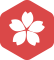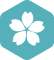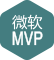### 高分求取C# contour组件及其画等高线用法...

.NET技术 > C# [问题点数：100分，结帖人stepman]2005年4月 VB大版内专家分月排行榜第一
2005年2月 VB大版内专家分月排行榜第一2004年10月 VB大版内专家分月排行榜第二stepman

matlab中contour 函数的用法（绘制等高线

matplotlib函数库使用contour绘制等高线图形

#coding=utf-8 import numpy as np import matplotlib.pyplot as plt def height(x,y): return (1-x/2+x**5+y**3)*np.exp(-x**2-y**2) x=np.linspace(-3,3,300) y=np.linspace(-3,3,300) X,Y=np.meshgrid(x,y) ...

matplotlib 等高线的绘制 —— plt.contour 与 plt.contourf

contour：轮廓，等高线。 为等高线上注明等高线的含义：cs = plt.contour(x, y, z) plt.clabel(cs, inline=1, fontsize=10) plt.contourf 与 plt.contour 区别：f：filled，也即对等高线间的填充区域进行填充（使用...

matlab 绘制等高线

contour 函数详解

contour用来绘制矩阵数据的等高线用法contour(Z) ：绘制矩阵Z的等高线 contour(Z,n) ：设置等高线的根数（出来有n根等高线contour(Z,v) ：v是元素随下标单调递增的一维向量，用来设置等高线的值。即在...

matlab绘制等高线

MATLAB提供函数contour（）绘制等高线图，函数contourf（）绘制经过填充的等高线图，具体调用格式如下：★ contour（z）：该函数绘制矩阵z的等高线。★ contour（x，y，z）：该函数在指定坐标（x，y）下，出矩阵 z...

matlab中的contour画曲面的等高线图 和pie 饼状图 surf三维图

【MATLAB】contour函数的使用（绘制等高线的深入讲解）

１.contour(Z) 创建一个包含矩阵 Z 的等值线的等高线图， 　其中 Z 包含 x-y 平面上的高度值。 　MATLAB® 会自动选择要显示的等高线。 　Z 的行索引和列索引分别是平面中的 x 和 y 坐标。 　２.contour(X,Y,Z...

MATLAB使用surf、contour3灵活绘制投影图、等高线图，显示在指定高度平面

【MATLAB】MATLAB 可视化之等高线

contour: 矩阵的等高线图 contourf: 填充的等高线图 contourc : 低级等高线contour3 : 3D等高线图 contourslice : 在体切片平面中绘制等高线 clabel : 为等高线添加高度标签 fcontour : 绘制函数的等高线 ...

python matplotlib contour画等高线

matplotlib学习—画等高线

matplotlib学习记录：简单的等高线

Python画图初体验----曲面图和等高线

import numpy as np import matplotlib.pyplot as plt from mpl_toolkits.mplot3d import Axes3D fig = plt.figure() ax = Axes3D(fig) #生成一个3d对象 X = np.arange(-4, 4, 0.25) Y = np.arange(-4, 4, 0.25) ...

Python可视化库matplotlib.pyplot里contour与contourf的区别

contour和contourf都是三维等高线图的，不同点在于contourf会对等高线间的区域进行填充，区别如下： import numpy as np import matplotlib.pyplot as plt from matplotlib.colors import ListedColormap x=np....

matplotlib的基本用法(九)——绘制等高线

matplotlib的基本用法(九)——绘制等高线

contourf()绘制填充颜色的二维等高线

contourf(Z)%等高线自动选择 contourf(Z,n)%n条线 contourf(Z,v)%在向量v的每个元素数值的位置绘制等高线 contourf(X,Y,Z)%指定Z中第i个元素的坐标为（X(i),Y(i)） contourf(X,Y,Z,n) contourf(X,Y,Z,v) contourf(.....

Matplotlib数据可视化——contour等高线图

Matlab中绘制网格图和等高线：mesh 和 surf

﻿﻿ 转自：http://huzhyi21.blog.163.com/blog/static/1007396201061052214302/ %author：huzhyi，2010-07-10 Matlab中绘制网状图、曲面图：mesh 和 surf x=linspace(-2, 2, 25); ...在x轴上25点 ...在y轴上2

【 MATLAB 】Contour plot of matrix（矩阵的等高线图）

contour(Z) 绘制矩阵Z的等高线图，其中Z被解释为相对于x-y平面的高度。 Z必须至少是包含至少两个不同值的2乘2的矩阵。 x值对应于Z的列索引，y值对应于Z的行索引。自动选择轮廓级别。 contour(Z,n) 绘制具有n个...

plt.contour

surfer10与Arcgis10.3 完美生成等值线和等值面方法

surfer10 与Arcgis10.3生成等值线和等值面的步骤如下： 1.准备surfer用的Book1.dat文件，格式如下： "x" "y" "z" 116.471992 40.809975 232 116.335556 40.700542 1235 116.541626 40.713333 214 116.73349 40....

matplotlib.pyplot contourf()函数的使用

python等高线图的画法

MATLAB绘制三维曲面图和等高线 绘图（2）

matlab contour 等高线返回每点坐标？

C is a two-row matrix specifying all the contour lines. Each contour line defined in matrix C begins with a column that contains the value of the contour (specified by v and used by clabel), and the n

Python Matplotlib contour和contourf：绘制等高线

MATLAB 中contour的应用

contour函数（绘制矩阵的等高线图） 1、contour(Z)绘制包含矩阵Z的等值线的等高线，自动选择等高线的层级 2、contour(X,Y,Z)指定X,Y的坐标 例如：x = linspace(-2*pi,2*pi); y = linspace(0,4*pi); [X,Y] = ...

python绘制等高线

# encoding: utf-8 import numpy as np import pandas as pd import matplotlib.pyplot as plt from mpl_toolkits import mplot3d fig=plt.figure() x_line=np.linspace(-6,6,30) y_line=np.linspace(-6,6,30) ...

8.7 密度和等高线图 原文：Density and Contour Plots 译者：飞龙 协议：CC BY-NC-SA 4.0 本节是《Python 数据科学手册》（Python Data Science Handbook）的摘录。 有时，使用等高线或颜色编码的区域，在二维中...